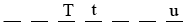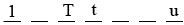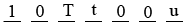SEARCH HOMEMath Central Quandaries & QueriesQuestion from Godryk, a student: I am the smallest 7-digit odd number. my ones digit is the sum of my thousands, and ten thousands digits. What number am I?Godryk,

Here is what the number will look like. I put u for the units digit (the ones digit), t for the thousands digit and T for the ten thousands digit.Since this is a 7 digit number the leading digit can't be zero. I want the number to be as small as possible so the leading digit must be 1.The only other requirements involve T, t and u so I can fill in the remaining 3 digits any way I want but keeping the number as small as possible. Thus the 3 remaining digits must be 0.The number is odd so u must be odd.

Can you complete the number?

PennyMath Central is supported by the University of Regina and The Pacific Institute for the Mathematical Sciences.